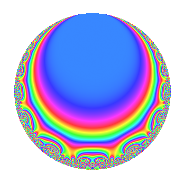# Properties

 Label 30.3.bLevel 30 Weight 3 Character orbit b Rep. character $$\chi_{30}(29,\cdot)$$ Character field $$\Q$$ Dimension 4 Newforms 1 Sturm bound 18 Trace bound 0

# Related objects

## Defining parameters

 Level: $$N$$ = $$30 = 2 \cdot 3 \cdot 5$$ Weight: $$k$$ = $$3$$ Character orbit: $$[\chi]$$ = 30.b (of order $$2$$ and degree $$1$$) Character conductor: $$\operatorname{cond}(\chi)$$ = $$15$$ Character field: $$\Q$$ Newforms: $$1$$ Sturm bound: $$18$$ Trace bound: $$0$$

## Dimensions

The following table gives the dimensions of various subspaces of $$M_{3}(30, [\chi])$$.

Total New Old
Modular forms 16 4 12
Cusp forms 8 4 4
Eisenstein series 8 0 8

## Trace form

 $$4q$$ $$\mathstrut +\mathstrut 8q^{4}$$ $$\mathstrut -\mathstrut 4q^{6}$$ $$\mathstrut -\mathstrut 32q^{9}$$ $$\mathstrut +\mathstrut O(q^{10})$$ $$4q$$ $$\mathstrut +\mathstrut 8q^{4}$$ $$\mathstrut -\mathstrut 4q^{6}$$ $$\mathstrut -\mathstrut 32q^{9}$$ $$\mathstrut -\mathstrut 16q^{10}$$ $$\mathstrut +\mathstrut 8q^{15}$$ $$\mathstrut +\mathstrut 16q^{16}$$ $$\mathstrut +\mathstrut 48q^{19}$$ $$\mathstrut +\mathstrut 68q^{21}$$ $$\mathstrut -\mathstrut 8q^{24}$$ $$\mathstrut -\mathstrut 36q^{25}$$ $$\mathstrut +\mathstrut 68q^{30}$$ $$\mathstrut -\mathstrut 128q^{31}$$ $$\mathstrut -\mathstrut 64q^{34}$$ $$\mathstrut -\mathstrut 64q^{36}$$ $$\mathstrut -\mathstrut 32q^{40}$$ $$\mathstrut -\mathstrut 68q^{45}$$ $$\mathstrut +\mathstrut 136q^{46}$$ $$\mathstrut +\mathstrut 60q^{49}$$ $$\mathstrut +\mathstrut 32q^{51}$$ $$\mathstrut +\mathstrut 100q^{54}$$ $$\mathstrut +\mathstrut 272q^{55}$$ $$\mathstrut +\mathstrut 16q^{60}$$ $$\mathstrut -\mathstrut 64q^{61}$$ $$\mathstrut +\mathstrut 32q^{64}$$ $$\mathstrut -\mathstrut 272q^{66}$$ $$\mathstrut -\mathstrut 68q^{69}$$ $$\mathstrut -\mathstrut 136q^{70}$$ $$\mathstrut -\mathstrut 272q^{75}$$ $$\mathstrut +\mathstrut 96q^{76}$$ $$\mathstrut -\mathstrut 288q^{79}$$ $$\mathstrut +\mathstrut 188q^{81}$$ $$\mathstrut +\mathstrut 136q^{84}$$ $$\mathstrut +\mathstrut 128q^{85}$$ $$\mathstrut +\mathstrut 128q^{90}$$ $$\mathstrut -\mathstrut 200q^{94}$$ $$\mathstrut -\mathstrut 16q^{96}$$ $$\mathstrut +\mathstrut 272q^{99}$$ $$\mathstrut +\mathstrut O(q^{100})$$

## Decomposition of $$S_{3}^{\mathrm{new}}(30, [\chi])$$ into irreducible Hecke orbits

Label Dim. $$A$$ Field CM Traces $q$-expansion
$$a_2$$ $$a_3$$ $$a_5$$ $$a_7$$
30.3.b.a $$4$$ $$0.817$$ $$\Q(\sqrt{2}, \sqrt{-17})$$ None $$0$$ $$0$$ $$0$$ $$0$$ $$q+\beta _{2}q^{2}+(-\beta _{1}-\beta _{2})q^{3}+2q^{4}+(-2\beta _{2}+\cdots)q^{5}+\cdots$$

## Decomposition of $$S_{3}^{\mathrm{old}}(30, [\chi])$$ into lower level spaces

$$S_{3}^{\mathrm{old}}(30, [\chi]) \cong$$ $$S_{3}^{\mathrm{new}}(15, [\chi])$$$$^{\oplus 2}$$